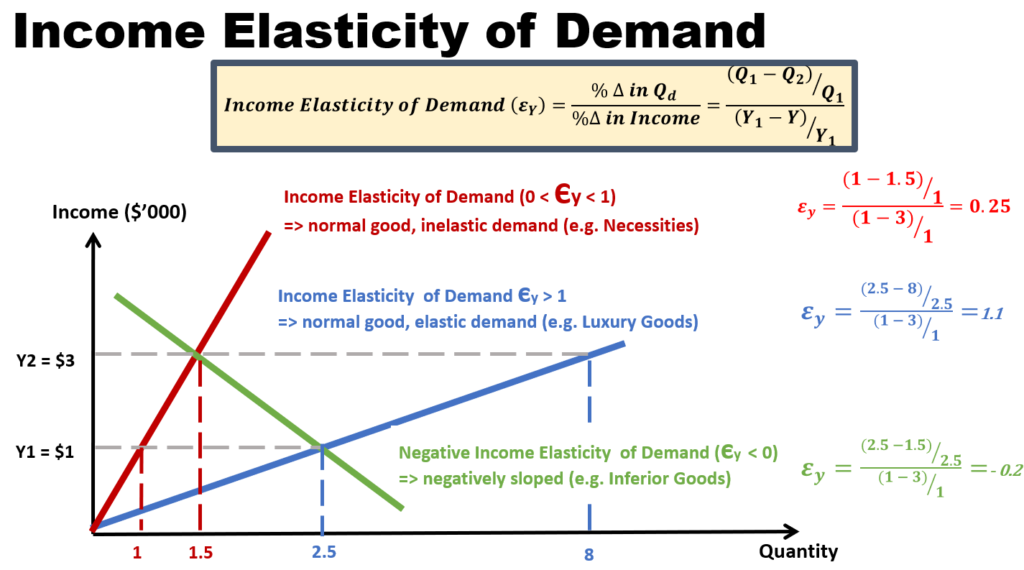# Income Elasticity of Demand

## How much big is the change in quantity over the change in income

Income elasticity of demand (Ey) is the degree of responsiveness of demand to changes in the income of consumers.

The formula for Ey is as shown in the diagram. The numerator is the percentage change in quantity demanded. This means that we take the change in quantity demanded over the base quantity demanded (i.e. original quantity demanded). The denominator is the percentage change in income. This means that we take the change in income over the base income (i.e. original income).

An alternative formula to calculate Ey is to substitute the base quantity demanded and base income with their average counterparts.

The sign of the Ey matters (unlike the case for price elasticity of demand).

• If Ey is between 0 and 1, then it is a normal good with inelastic demand, such as a necessity good like food.
• If Ey is greater than 1, then it is a normal good with elastic demand, such as a luxury good like an expensive watch.
• If Ey is below 0, then it is an inferior good such as cheap beer, and low grade rice.Based on the size of Ey and whether it is positive or negative, we can learn more about the type of goods the producer is selling. The converse is also true. That is, if we know the type of goods that the producer is selling, we can predict what will happen if income of consumer increases or falls.